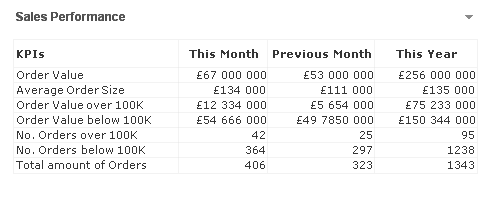# Qlik Design Blog

All about product and Qlik solutions: scripting, data modeling, visual design, extensions, best practices, etc.Employee

## ValueList() – For those tricky situations

Have you ever been asked to create a table that has several independent calculations over different metrics?

Mixing aggregation formulas and counts in the same table?

Did you end up creating different tables for every view point on the same information?

Or did you create a table like the one below?If not, let me introduce you to ValueList() and its number oriented big brother ValueLoop().

## ValueListValueList (value {, value })

ValueList allows us to specify a set of arbitrary values within the function, when used as a calculated dimension in a chart this will act as a synthetic dimension.

We can later restate the same function, with the same parameters, in our expression to reference the corresponding value in our newly created synthetic dimension.

And it is as simple as creating a straight table with following dimension and expression

Calculated Dimension:

`=ValueList('My First KPI','My Second KPI')`

Expression:

`=IF( ValueList('My First KPI','My Second KPI')='My First KPI',      Sum([My First KPI Field],      Count([My Second KPI Field]))`

And voila we have created a table/chart with a dimension that does not exist in our data model and with an expression that has the possibility to mix and match aggregation functions over each dimension.

Matthew Crowther has also created an excellent Explosion Chart that also leverages ValueList, you can read more on his blog

## ValueLoopValueLoop(from [, to [, step = 1 ]])

ValueLoop shares the same characteristics as it’s little brother ValueList with the exception that it will create a series of numbers as the synthetic dimension.

To create a dimension with values that spans between 1-100 we would create a calculated dimension with

`=ValueLoop(1,100,1) `

which we can reference from our expression with the expression

`IF( ValueLoop(1,100,1)=3,     'Almost Pi',     'Not Pi')`

ValueLoop also allows us to create the, not so useful but fun to make, square pie chart which you can read more on in this technical brief.Creator

Yes Alexis,

Use NODISTINCT as follows:

pick(match(valuelist('A','B','C')

'A','B','C')

,sum(aggr(NODISTINCT if((min({<planyear_endyear={\$(=max(planyear_endyear)-1)}>} salesfigure)) > 10000, 1,0), storeID))

,sum(aggr(NODISTINCT if((min({<planyear_endyear={\$(=max(planyear_endyear)-1)}>} salesfigure)) < 10000, 1,0), storeID))

,sum(aggr(NODISTINCT if((min({<planyear_endyear={\$(=max(planyear_endyear)-1)}>} salesfigure)) = 0, 1,0), storeID))

)

Thanks.

8,212 ViewsNot applicable

It works!, Thanks very much.

Alexis

8,212 ViewsContributor

Is there a way to have the value list items be conditional expressions. For example, I have a value list that right now is current month, previous month, 2 months ago.

Instead I want them to be Month(Max(RT_Date)), etc. So based on the latest inputs the dimensions will show as May or whatever the months are.

Is this possible?

8,212 ViewsCreator III

Hi Nick -

Yup.... I did it user enterable values. Basically, you use variables in your valuelist statement. Something like:

ValueList('\$(vStage1)',

'\$(vStage2)',

'\$(vStage3)',

'\$(vStage4)',

'\$(vStage5)',

' Total Open')

I have a post on my personal blog if you can stand to read through my thought process...

Fortune Cookie BI: Perfect Probability Pipeline

mike

8,212 ViewsPartner

Hello guys, amazing article!

However, it still doesn't fully solve my issue as I am having more than 100 KPIs that the user requires to be able to dynamically choose form. Therefore, I need to list them all in a dimension in a straight table with a valuelist() and use that valueliest() in a pick(match()) or nested Ifs as an expression. All of this takes ages to calculate. Any ideas?

2,892 Views